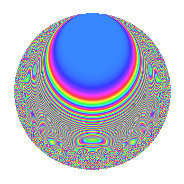# Properties

 Label 8015.2.a.mLevel 8015 Weight 2 Character orbit 8015.a Self dual Yes Analytic conductor 64.000 Analytic rank 0 Dimension 67 CM No

# Related objects

## Newspace parameters

 Level: $$N$$ = $$8015 = 5 \cdot 7 \cdot 229$$ Weight: $$k$$ = $$2$$ Character orbit: $$[\chi]$$ = 8015.a (trivial)

## Newform invariants

 Self dual: Yes Analytic conductor: $$64.0000972201$$ Analytic rank: $$0$$ Dimension: $$67$$ Fricke sign: $$-1$$ Sato-Tate group: $\mathrm{SU}(2)$

## $q$-expansion

The dimension is sufficiently large that we do not compute an algebraic $$q$$-expansion, but we have computed the trace expansion.

 $$\operatorname{Tr}(f)(q) =$$ $$67q$$ $$\mathstrut +\mathstrut 3q^{2}$$ $$\mathstrut +\mathstrut 73q^{4}$$ $$\mathstrut +\mathstrut 67q^{5}$$ $$\mathstrut +\mathstrut 17q^{6}$$ $$\mathstrut -\mathstrut 67q^{7}$$ $$\mathstrut +\mathstrut 12q^{8}$$ $$\mathstrut +\mathstrut 97q^{9}$$ $$\mathstrut +\mathstrut O(q^{10})$$ $$\operatorname{Tr}(f)(q) =$$ $$67q$$ $$\mathstrut +\mathstrut 3q^{2}$$ $$\mathstrut +\mathstrut 73q^{4}$$ $$\mathstrut +\mathstrut 67q^{5}$$ $$\mathstrut +\mathstrut 17q^{6}$$ $$\mathstrut -\mathstrut 67q^{7}$$ $$\mathstrut +\mathstrut 12q^{8}$$ $$\mathstrut +\mathstrut 97q^{9}$$ $$\mathstrut +\mathstrut 3q^{10}$$ $$\mathstrut +\mathstrut 11q^{11}$$ $$\mathstrut +\mathstrut 9q^{12}$$ $$\mathstrut +\mathstrut 19q^{13}$$ $$\mathstrut -\mathstrut 3q^{14}$$ $$\mathstrut +\mathstrut 93q^{16}$$ $$\mathstrut +\mathstrut 7q^{17}$$ $$\mathstrut +\mathstrut 9q^{18}$$ $$\mathstrut +\mathstrut 36q^{19}$$ $$\mathstrut +\mathstrut 73q^{20}$$ $$\mathstrut -\mathstrut 8q^{22}$$ $$\mathstrut -\mathstrut 2q^{23}$$ $$\mathstrut +\mathstrut 37q^{24}$$ $$\mathstrut +\mathstrut 67q^{25}$$ $$\mathstrut +\mathstrut 39q^{26}$$ $$\mathstrut +\mathstrut 6q^{27}$$ $$\mathstrut -\mathstrut 73q^{28}$$ $$\mathstrut +\mathstrut 56q^{29}$$ $$\mathstrut +\mathstrut 17q^{30}$$ $$\mathstrut +\mathstrut 63q^{31}$$ $$\mathstrut +\mathstrut 27q^{32}$$ $$\mathstrut +\mathstrut 51q^{33}$$ $$\mathstrut +\mathstrut 55q^{34}$$ $$\mathstrut -\mathstrut 67q^{35}$$ $$\mathstrut +\mathstrut 148q^{36}$$ $$\mathstrut +\mathstrut 28q^{37}$$ $$\mathstrut +\mathstrut 10q^{38}$$ $$\mathstrut +\mathstrut 7q^{39}$$ $$\mathstrut +\mathstrut 12q^{40}$$ $$\mathstrut +\mathstrut 84q^{41}$$ $$\mathstrut -\mathstrut 17q^{42}$$ $$\mathstrut -\mathstrut 11q^{43}$$ $$\mathstrut +\mathstrut 41q^{44}$$ $$\mathstrut +\mathstrut 97q^{45}$$ $$\mathstrut +\mathstrut 17q^{46}$$ $$\mathstrut -\mathstrut 10q^{47}$$ $$\mathstrut +\mathstrut 10q^{48}$$ $$\mathstrut +\mathstrut 67q^{49}$$ $$\mathstrut +\mathstrut 3q^{50}$$ $$\mathstrut -\mathstrut q^{51}$$ $$\mathstrut +\mathstrut 49q^{52}$$ $$\mathstrut +\mathstrut 3q^{53}$$ $$\mathstrut +\mathstrut 20q^{54}$$ $$\mathstrut +\mathstrut 11q^{55}$$ $$\mathstrut -\mathstrut 12q^{56}$$ $$\mathstrut +\mathstrut 45q^{57}$$ $$\mathstrut +\mathstrut 22q^{58}$$ $$\mathstrut +\mathstrut 80q^{59}$$ $$\mathstrut +\mathstrut 9q^{60}$$ $$\mathstrut +\mathstrut 64q^{61}$$ $$\mathstrut -\mathstrut 37q^{62}$$ $$\mathstrut -\mathstrut 97q^{63}$$ $$\mathstrut +\mathstrut 110q^{64}$$ $$\mathstrut +\mathstrut 19q^{65}$$ $$\mathstrut +\mathstrut 75q^{66}$$ $$\mathstrut +\mathstrut 22q^{67}$$ $$\mathstrut +\mathstrut 7q^{68}$$ $$\mathstrut +\mathstrut 107q^{69}$$ $$\mathstrut -\mathstrut 3q^{70}$$ $$\mathstrut +\mathstrut 24q^{71}$$ $$\mathstrut +\mathstrut 72q^{72}$$ $$\mathstrut +\mathstrut 83q^{73}$$ $$\mathstrut +\mathstrut 52q^{74}$$ $$\mathstrut +\mathstrut 115q^{76}$$ $$\mathstrut -\mathstrut 11q^{77}$$ $$\mathstrut -\mathstrut 70q^{78}$$ $$\mathstrut -\mathstrut 32q^{79}$$ $$\mathstrut +\mathstrut 93q^{80}$$ $$\mathstrut +\mathstrut 183q^{81}$$ $$\mathstrut +\mathstrut 56q^{82}$$ $$\mathstrut -\mathstrut 58q^{83}$$ $$\mathstrut -\mathstrut 9q^{84}$$ $$\mathstrut +\mathstrut 7q^{85}$$ $$\mathstrut +\mathstrut 51q^{86}$$ $$\mathstrut +\mathstrut 20q^{87}$$ $$\mathstrut -\mathstrut 5q^{88}$$ $$\mathstrut +\mathstrut 129q^{89}$$ $$\mathstrut +\mathstrut 9q^{90}$$ $$\mathstrut -\mathstrut 19q^{91}$$ $$\mathstrut -\mathstrut 37q^{92}$$ $$\mathstrut +\mathstrut 33q^{93}$$ $$\mathstrut +\mathstrut 89q^{94}$$ $$\mathstrut +\mathstrut 36q^{95}$$ $$\mathstrut +\mathstrut 129q^{96}$$ $$\mathstrut +\mathstrut 126q^{97}$$ $$\mathstrut +\mathstrut 3q^{98}$$ $$\mathstrut -\mathstrut 27q^{99}$$ $$\mathstrut +\mathstrut O(q^{100})$$

## Embeddings

For each embedding $$\iota_m$$ of the coefficient field, the values $$\iota_m(a_n)$$ are shown below.

For more information on an embedded modular form you can click on its label.

Label $$a_{2}$$ $$a_{3}$$ $$a_{4}$$ $$a_{5}$$ $$a_{6}$$ $$a_{7}$$ $$a_{8}$$ $$a_{9}$$ $$a_{10}$$
1.1 −2.79998 −2.08197 5.83989 1.00000 5.82947 −1.00000 −10.7516 1.33460 −2.79998
1.2 −2.72902 1.60790 5.44753 1.00000 −4.38799 −1.00000 −9.40835 −0.414653 −2.72902
1.3 −2.66598 2.60914 5.10744 1.00000 −6.95591 −1.00000 −8.28438 3.80761 −2.66598
1.4 −2.55168 −1.07267 4.51109 1.00000 2.73711 −1.00000 −6.40752 −1.84938 −2.55168
1.5 −2.54658 −2.85949 4.48508 1.00000 7.28192 −1.00000 −6.32845 5.17667 −2.54658
1.6 −2.53656 −2.78475 4.43415 1.00000 7.06368 −1.00000 −6.17438 4.75481 −2.53656
1.7 −2.40339 −1.71467 3.77630 1.00000 4.12102 −1.00000 −4.26915 −0.0599106 −2.40339
1.8 −2.30662 1.34001 3.32049 1.00000 −3.09089 −1.00000 −3.04587 −1.20437 −2.30662
1.9 −2.25277 3.29965 3.07495 1.00000 −7.43334 −1.00000 −2.42162 7.88769 −2.25277
1.10 −2.05677 1.58909 2.23031 1.00000 −3.26839 −1.00000 −0.473688 −0.474802 −2.05677
1.11 −1.94503 2.18285 1.78313 1.00000 −4.24571 −1.00000 0.421811 1.76484 −1.94503
1.12 −1.89706 −1.32001 1.59885 1.00000 2.50414 −1.00000 0.761013 −1.25758 −1.89706
1.13 −1.83785 1.36680 1.37770 1.00000 −2.51199 −1.00000 1.14369 −1.13184 −1.83785
1.14 −1.82956 −1.24341 1.34731 1.00000 2.27489 −1.00000 1.19414 −1.45394 −1.82956
1.15 −1.80341 0.567965 1.25230 1.00000 −1.02428 −1.00000 1.34841 −2.67742 −1.80341
1.16 −1.76232 −3.28691 1.10578 1.00000 5.79259 −1.00000 1.57590 7.80377 −1.76232
1.17 −1.76209 −0.304110 1.10496 1.00000 0.535869 −1.00000 1.57714 −2.90752 −1.76209
1.18 −1.50021 0.266806 0.250644 1.00000 −0.400267 −1.00000 2.62441 −2.92881 −1.50021
1.19 −1.44039 2.88342 0.0747197 1.00000 −4.15325 −1.00000 2.77315 5.31413 −1.44039
1.20 −1.42994 −3.33526 0.0447232 1.00000 4.76921 −1.00000 2.79592 8.12394 −1.42994
See all 67 embeddings
 $$n$$: e.g. 2-40 or 990-1000 Embeddings: e.g. 1-3 or 1.67 Significant digits: Format: Complex embeddings Normalized embeddings Satake parameters Satake angles

## Inner twists

This newform does not have CM; other inner twists have not been computed.

## Atkin-Lehner signs

$$p$$ Sign
$$5$$ $$-1$$
$$7$$ $$1$$
$$229$$ $$1$$

## Hecke kernels

This newform can be constructed as the intersection of the kernels of the following linear operators acting on $$S_{2}^{\mathrm{new}}(\Gamma_0(8015))$$:

 $$T_{2}^{67} - \cdots$$ $$T_{3}^{67} - \cdots$$